MORE IN Engineering Mechanics
MU First Year Engineering (Semester 1)
Engineering Mechanics
May 2012
Total marks: --
Total time: --
INSTRUCTIONS
(1) Assume appropriate data and state your reasons
(2) Marks are given to the right of every question
(3) Draw neat diagrams wherever necessary

1 (a) The resultant of the there foreces shown in figure and other forces two forces. P and Q acting at 'A' and 'B' is a couple magnitude 120 kNm clockwise. Determine the force P and Q.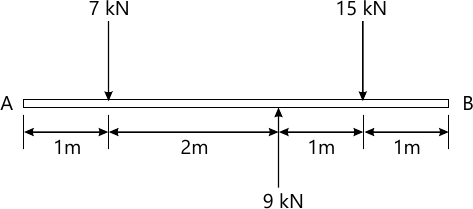5 M
1 (b)

A thin homogeneous wire of uniform section is built into a shape as shown in figure. Determine the position of c.g. of a wire. Take ? =30? and r=15cm.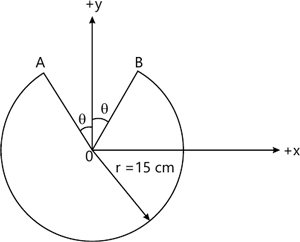5 M
1 (c) During a test, the car, moves in a straight line such that its velocity is defined by v=0.3 (9t2 + 2t) where 't' is in seconds. Determine the position and acceleration when t=3 sec. take at t=0, x=0.
5 M
1 (d) A man in a balloon of 2 m/s relative to the balloon. After what time interval will the ball return to the balloon.
5 M
1 (e) A point moves along a curved path y=0.4x2, At x=2m its speed is 6 m/s increasing at 3 m/s2. At this instatnt find- (i) Velocity components along x and y direction. (ii) Its acceleration.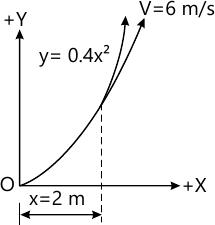5 M
1 (f) State and prove work Energy principle.
5 M

2 (a) Find the reactions at 'A'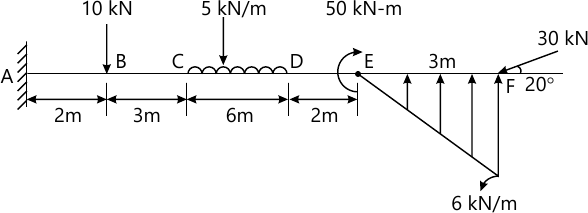10 M
2 (b) Three cylinders are pilled up in a rectangular channel as shown in figure. Determine the reaction between cylinders A,B and C with channel surfaces. Assume all smooth surface.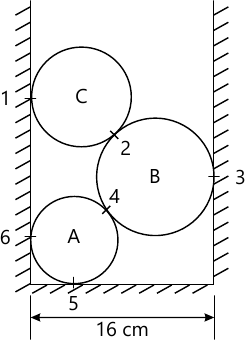WA=150 N, RA=4 cm

WB=400 N, RB=6 cm

WC=200 N, RC=5 cm

10 M

3 (a) Determine forces in all members of the plane truss as shown in figure.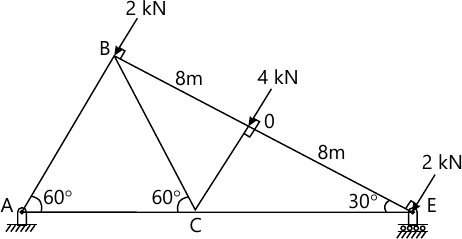10 M
3 (b) Two spheres rests on a smooth surfaces as shown in figure. Find the reaction at points of contact 1,2,3, and 4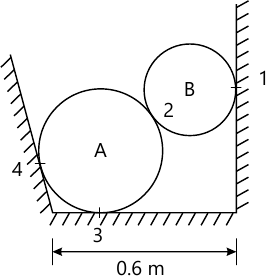WA= 500 N, WB=200 N,

RA=0.25m, RB=0.20m

10 M

4 (a) Two blocks A and B are resting against a wal the floor as shown in figure. Find horizontal forces 'P' applied to the lower block that will hold the system in equilibrium. Take ?=0.25 at floor, ?=0.3 at wall and ?=0.2 between the blocks WA=500 N, We=1000 N.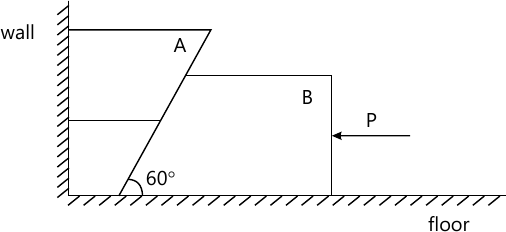10 M
4 (b) Find moment of Inertia of the shaded are as shown in figure about its references x and y axls.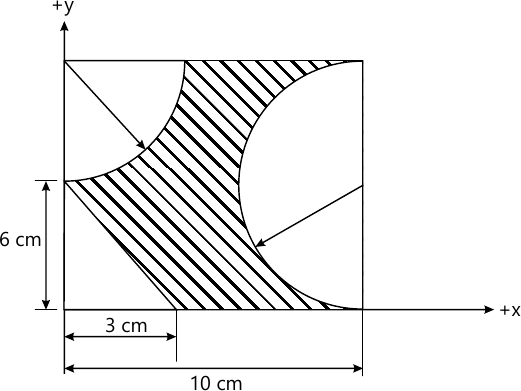10 M

5 (a) A train leaves station 'A' and attains speed at the rate of 4 m/s2 for 6 sec and then 6 m/s2 till it reaches a velocity of 48 m/s. Further the velocity remains constatnt, then brakes are applied giving the train a constant deacceleration stopping it in 6 sec. If the total running time between two stations is 40 sec. plot a-t, v-t and x-t curve. Determine distance between two stations.
10 M
5 (b) A spring is used to stop 100 kg package which is moving down a 30? incline. The spring has constant k=30 kN/m is held by cables so that it is initially compressed by 90 mm. If the velocities of package is 5 m/s, when it is 9 m from spring. Determine the maximum additional deformation of spring in bringing the package to rest. Assume coefficient between block and incline as 0.2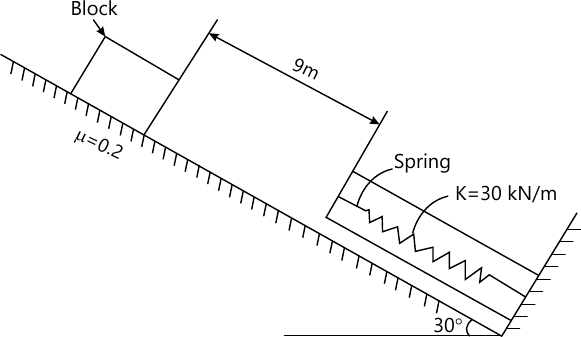10 M

6 (a) Two smooth balls collides as shown in figure. If mA= 1 kg, mB=2kg and e= 0.75 Find the velocities after impact.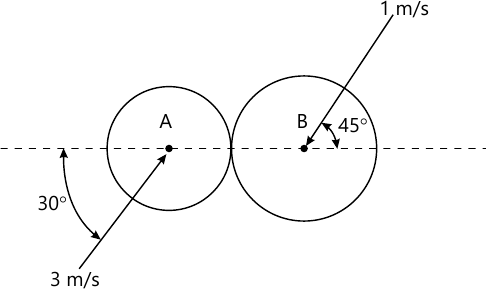10 M
6 (b) A ball throw down the incline strike the incline at adistance of 75 m along. If the ball rises 20 m above the point of projection. Complete the initial velocity and angle of projection with horizontal.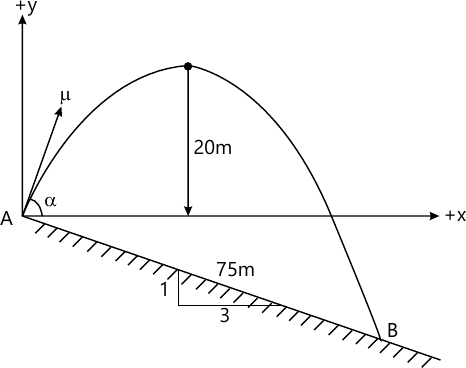10 M

7 (a) The system shown in figure is released from rest. What is the height lost by bodies A, B and C in 2 seconds? Take coefficient of kinetic friction at rubbing surface as 0.4. Find also the tension in wires.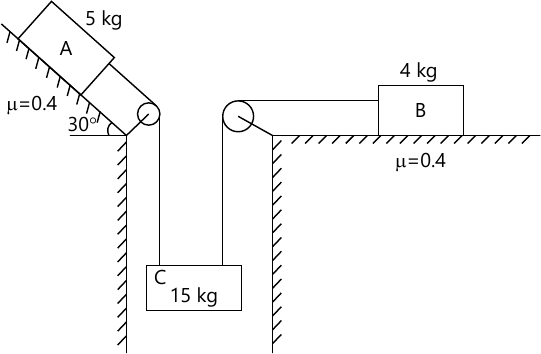10 M
7 (b) For the link and slider mechanism shown in figure, locate the instantaneous centre of rotation of link AB. Also find the angular velocity of link 'OA'. Take velocity of slider at B=2500 mm/sec.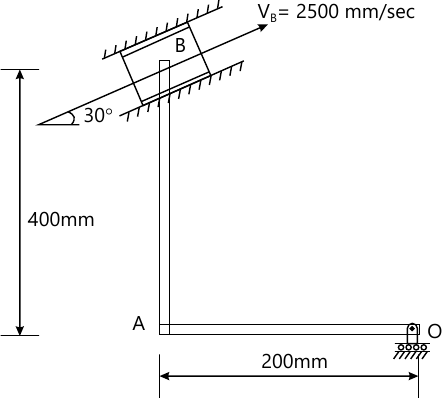10 M

More question papers from Engineering Mechanics